#### Inspiration:

There is a game played a while back that I really liked, and as recreating that game will take much longer than the time I have now, I got an idea that is similar in a way, but simpler.

The game I got inspired by is called HUE.In this game, hue needs to save his mom by collecting pieces of the color ring in the picture above.

#### My Idea:

My idea, similarly, the student needs to collect a few questions and the exam to pass.

Game Parts:

– The player/student needs to collect all the questions on the screen and get to the final before the timer ends.

– If the student collects all the questions, they can collect the final exam paper and get the full grade on the exam. Otherwise, they get points for the questions they collected.

-each question is guarded by a monster(monsters are inspired by the monsters that face students like anxiety, procrastination, and distractions).

This is a rough draft of what the game is supposed to look like: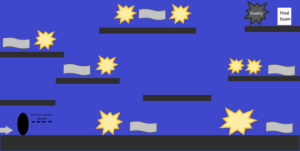#### Progress:

I started with the background. Instead of using a still image, I used loadPixels() to make the background look something like a night sky.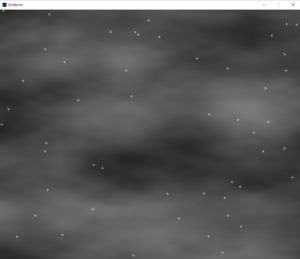##### Code:

```class Bg {
float[] x = new float;
float[] y = new float;
Bg() {
for (int i=0; i<50; i++) {
x[i] = random(0, widthh);
y[i] = random(0, heightt);
}
}
void drawBG() {
for (int y=0; y<heightt; y++) {
for (int x=0; x<widthh; x++) {
float n = noise(x*.002, y*.005);
//n = frameCount%width+n;
//n= map(n,0,width,0,255);
pixels[x+y*width]=color(n*150);
}
}
updatePixels();

if (frameCount%5==0) {
for (int i=0; i<50; i++) {
x[i] = random(0, width);
y[i] = random(0, height);
}
}
for (int i=0; i<50; i++) {
for (int j=0; j<10; j++) {
noStroke();
fill(255, 25);
ellipse(x[i], y[i], 1+j, 1+j);
}
}
}
}
```

I added platforms, which the questions and monsters will be on at random, saved, x-locations: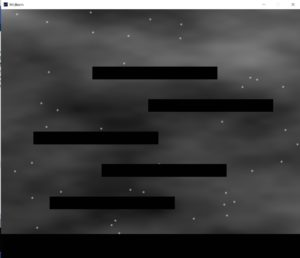##### Code:
```class Platforms {

PVector pos; //mid point
float pHeight;
float pWidth;
float widths;

Platforms(float x, float y, float w, float h) {
pos = new PVector(x, y);
//println(pos.x, pos.y);
pHeight = h;
pWidth = w;
}

void display() {
stroke(0);
//noStroke();
rectMode(CORNER);
fill(1);
rect(pos.x, pos.y, pWidth, pHeight);
}
// float distance(Objects other){

// }
}```

My code is mainly classes for each thing on the screen and one for the whole game that controls everything.

I made one creature’s class that will be a superclass for the player, monsters, questions, and the final.

The class still has a few errors that I am working on.

```class Creature {

// Attributes
PVector pos;
PVector speed;
boolean alive;
float ground;
float size;
PVector imgSize;
int numFrames;
PImage img;
int frame;
boolean dir; //left = 0 right = 1

//Player
Creature(float x,float  y,float  r,float  g,float  img,float  w,float  h,int  num_frames,float  xl,float  xr) {
pos= new PVector(x,y);
speed=new PVector(0,0);
alive = true;
ground = g;
size = r;
imgSize = new PVector(w,h);
numFrames = num_frames;
frame = 0;
dir = true;
}

void gravity() {
if (pos.y+size >= ground) {
speed.y=0;
} else {
speed.y +=0.3;
if (pos.y+size+speed.y>ground) {
speed.y = ground-(pos.y+size);
}
}

for (int p=0; p<game.plats.length; p++) {
if (game.plats[p].pos.y + size <= game.plats[p].pos.y && pos.x+size>=game.plats[p].pos.x && pos.x-size <= game.plats[p].pos.x+game.plats[p].pos.x) {
ground = game.plats[p].pos.y;
break;
} else {
ground = game.ground;
}
}
}
void update(){
gravity();
pos.x += speed.x;
pos.y += speed.y;
}
void display(){
update();

if (dir && alive){
image(img, pos.x-imgSize.x/2 , pos.y-imgSize.y/2, frame*imgSize.x,0,(frame+1)*imgSize.x,imgSize,imgSize.y);
}
else if(alive){
image(img, pos.x-imgSize.x/2 , pos.y-imgSize.y/2, frame*imgSize.x,(frame+1)*imgSize.x,0,imgSize,imgSize.y);
}
}
}
void startSc(){

}

void endSc(){

}```

Finally, my game class and setup and draw functions that control the whole progress of the game.

the game being in a class allows me to easily restart the game if the player fails by calling a new game object again.

```class Game {

//
int ground = 100;

//   Player _player;
Platforms[] plats = new Platforms;
Platforms ground;
int platLocH[] = new int;
int platLocW[] = new int;

// Objects[] _monsters = new Objects;
// Objects _final;
// Objects[] _questions = new Objects;

// Bullets _bullets;

Game() {
ground = new Platforms(0, heightt-ground, widthh, ground);
for (int i=0; i<plats.length; i++) {
platLocH[i] = (heightt-250)-i*130;
platLocW[i] = int(random(0, widthh-500));
plats[i]= new Platforms(platLocW[i], platLocH[i], 500, heightt/20);
println((heightt)-i*150);
}
}

void main() {
for (int i = 0; i<5; i++) {
plats[i].display();
}
ground.display();
}
}

Bg bckgrnd = new Bg();
Game game = new Game();

void draw() {
bckgrnd.drawBG();

game.main();
//rect(height/2, width/2, 100,100);
}

void keyPressed() {
//   if (_start.getflag==True) {
//   }
}
```

#### Character Sprites:

These are my character sprites and draft sprites for the final exam and the questions: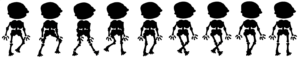Questions: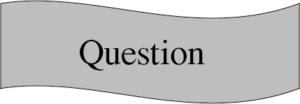## One thought on “Midterm Progress – deadStudent”

1.Aaron says:

Looks great Fatema. I don’t have much to suggest at this point game-wise. Nice use of making the background with noise. Doing the calculations for that background every frame is going to be quite expensive computationally. Look into using a PGraphics object (look it up in the reference) to draw that background scene into once during setup. then you can just draw the pgraphics object like an image and not have to do noise calculations every pixel every frame. You can also see an example of how a pgraphic works in the mask example from last week.